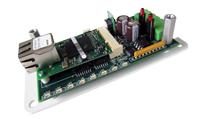# Creating Interval Data with the PMC-1

May 2017

 Often times the PMC-1 is called upon to create 15-minute interval data, within a client application program that uses the Modbus protocol.  15-Minute interval data is the standard format of the utility industry for load profiling and billing data.  For those not familiar with interval data, electric energy use (in kWh) is tracked usually over a 15-minute period.  This period is known as the meter interval or demand interval.  All metering uses one or more 15-minute intervals for tracking energy use and peak demand.  If a utility uses a demand interval of 30 or 60 minutes, multiple 15-minute periods are combined to accumulate energy use and calculate the correct peak demand over the selected interval. In the truest sense of the word, interval data was derived from counting KYZ pulses over a fixed 15-minute interval.  Intervals were started and stopped with an “End-Of-Interval” pulse on a second pulse channel of the meter.  These days most utilities are reluctant to supply EOI pulses to customer.  Therefore, to simulate the meter’s 15-minute intervals perfectly, the client software needs to establish an accurate time base, hopefully perfectly sync’d with the meter’s clock.  This is generally done by using an internet time server, polled at some recurring rate to correct a software real time clock running within the application or perhaps the PC’s hardware real time clock running the client Modbus software.  In any case, since most AMI electric meters perform an end-of-interval (EOI) operation on the quarter hour, the client software must perform reads of the Modbus register at precisely the same times:  00:00:00, 00:15:00, 00:30:00 and 00:45:00, for example. Using the PMC-1, the easiest, fastest and most accurate way to create interval data that will match the utility interval data is to use the Modbus register that holds the raw pulse count.  This has the highest probability of matching the utility meter’s exact pulse count.  Upon the clock striking the quarter hour mark, the client software initiates a read of the raw pulse count register for channel 1 or channel 2 (or both). Subtracting the last interval’s pulse count from the current interval’s pulse count will get you the NET pulse count.  Once the net pulse count has been captured, it is multiplied by the pulse constant or pulse value to get the energy used in the interval.   Using the correct pulse value requires knowing the difference between 2-wire (Form A) and 3-Wire (Form C) pulses.  If you selected “2-Wire” pulses (the default) in the setup of the PMC-1, then you are counting ONLY the closure of one side (K-Y) of the meter’s pulse initiator.  If you selected “3-Wire” pulses in the setup of the PMC-1, then you are counting BOTH the closure and the subsequent opening, which exactly emulates the legacy 3-Wire KYZ pulse metering standard.  In either case, you’ll get the correct answer if you apply the correct pulse constant.  For example, suppose the utility supplied you with a value of .072 kWh per pulse as the standard 3-wire (Form C) pulse value.  Use that value if you selected 3-Wire in the PMC-1 setup.  However, if you selected 2-Wire in the PMC-1 setup, then you must DOUBLE the value to .144 kWh per pulse to get the equivalent energy since you are only counting the closure and not the opening, that is, half the pulses of the 3-Wire method. Let’s assume that you’ve selected the 2-Wire mode, and thus in this example the pulse value is .144 kWh per pulse.  Let’s have our client software perform a register read at exactly the quarter hour.  In this example, let’s read the pulse count register.  Subtract this raw pulse count value from the last register count value read 15 minutes ago, yielding a net pulse count of 1754.  Multiply 1754 X .144 = 252.576 kilowatt-hours.  This is the Energy that was consumed in the prior interval.  Presumably, this energy value is saved in a database by the application program. The kW demand for the interval must by calculated by dividing the Energy by .25 hours (same as multiplying by 4).  In this case, the math is 252.576 kWh / .25 hours (since the 15 minute interval is ¼ of the hour) which equals 1,010.3 kilowatts.  This is the average demand for the interval.  You don’t know exactly how the instantaneous demand occurred from second to second, or minute to minute, to arrive at the average demand over that interval, but from a metering and billing standpoint, it does not matter.  The electric meter, and thus the billing rate, only cares about the average 15-minute demand.  Keep in mind that there are approximately 3,000 15-minute intervals in a typical 31 day month.  To determine the customer’s monthly peak demand, one of these intervals out of the 3,000 has the highest kWh use, and thus the highest demand. The highest average demand is the “Peak” demand, to which the utility’s peak demand charge is applied. Alternately, the pulse count register could be reset after each read, but that takes time and could possibly cause a missed incoming pulse to be counted, although the probability of this happening is astronomically small.  Nevertheless, the most reliable method of creating interval data from pulses is to let the pulse count register continue to climb until rollover, and then start over. For 30-minute or 60-minute demand intervals, you have two choices of how to do the register reads.  Use the method above or use a 30 or 60 minute time base.  Both methods will produce the same result. The PMC-1 gives you the flexibility to do it either way.PMC-1 Pulse Modbus Converter